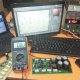Breaking News
Home / Electric / How to use a multimeter to calculate the Watts

# How to use a multimeter to calculate the Watts

## Using multimeter to calculate the Watts

Volts, Amps, and Watts Explained for the Water pump using amp meter:

We can see the picture. There have one AC Motor and a  Multi-function Digital Clamp Multimeter. We also call ampere meter. Normally we use this meter for repairing the Air condition and refrigerator. now we are going to calculate the Watts by using this Multimeter. How to use a multimeter?

.

## Today we are going to know how to calculate the Watts for any electronic goods.

normally we use the product but we don’t know how many Watts that product. here we can learn how to know the watts, ampere, and voltage.

For those who are using 220 AC Voltage

## 220 / 440 = 2 ampere

Normally we buy and use electronics and electric product. but we don’t follow the actual rules the using the product. for this sometime we make fire or unwanted situation. we use but we don’t follow the electric wire gauge. or the electric socket. for an example, an electric room heater. it’s 2000Watts. now I am going to use this in my room.

But normally in a room, our AC socket is not so heavy for using 2000Watts heater. for this, we must use heavy electric wire and socket otherwise the heater can make fire.

2000 / 220 =9.0 ampere. so if we want to use 2000 Watts room heater we need 9-ampere gauge wire and heavy electric socket. otherwise, the wire will make hot and it will make fire.

Which Country are using 110 AC Voltage

## 110 / 220 = 2 ampere

If you want to use 1000 Watts heater you need strong gauge wire and heavy-duty electric socket.

110 / 1000 = 9.0 ampere. if you use 1000 Watts, you must need 9.0-ampere wire. otherwise, the electric wire can make fire.

Now we are going to know how to calculate how many amperes need for your electric goods. here we can see the video by using an AC motor but you can use any electrical goods for calculating the Watts by using Multimeter.

let’s  Watch the Video this video can help you to calculate the Watts and ampere by using ampere meter.

You can use this ampere meter for any electric and electronic goods for calculating the ampere and watts.

For an example, you have an electric cooker but you don’t know that how many watts?

first, you switch on the cooker and use the meter as I use in this video. you get an ampere number.

like 3 ampere and your AC voltage is 110. so its so easy to calculate the Watts.

We know Voltage X Ampere = Watts.

So 110X3= 330 Watts.

Thanks for watching the video.if you like please like our Facebook Page

transistor circuit diagram of 2sa1943 and 2sc5200

Thanks a lot to be with us. If you want another post then please visit our website.

We have another post for you. like as repairing amplifier,